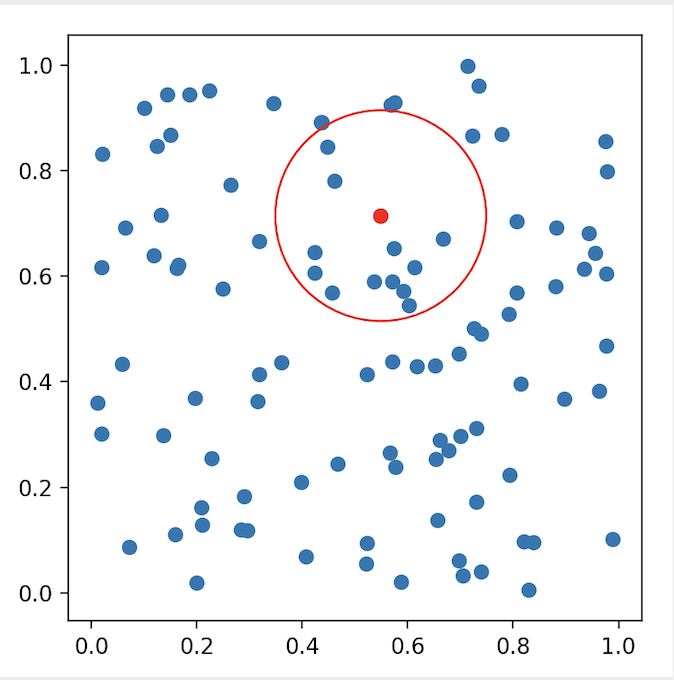## k-d tree算法原理及实现

k-d tree即k-dimensional tree，常用来作空间划分及近邻搜索，是二叉空间划分树的一个特例。通常，对于维度为$k$，数据点数为$N$的数据集，k-d tree适用于$N\gg2^k$的情形。

1）k-d tree算法原理

k-d tree是每个节点均为k维数值点的二叉树，其上的每个节点代表一个超平面，该超平面垂直于当前划分维度的坐标轴，并在该维度上将空间划分为两部分，一部分在其左子树，另一部分在其右子树。即若当前节点的划分维度为d，其左子树上所有点在d维的坐标值均小于当前值，右子树上所有点在d维的坐标值均大于等于当前值，本定义对其任意子节点均成立。

1.1）树的构建

a）切分维度选择优化

b）中值选择优化

a） 构建根节点时，此时的切分维度为$x$，如上点集合在$x$维从小到大排序为(2,3)，(4,7)，(5,4)，(7,2)，(8,1)，(9,6)；其中值为(7,2)。（注：2,4,5,7,8,9在数学中的中值为(5 + 7)/2=6，但因该算法的中值需在点集合之内，所以本文中值计算用的是len(points)//2=3, points=(7,2)）

b） (2,3)，(4,7)，(5,4)挂在(7,2)节点的左子树，(8,1)，(9,6)挂在(7,2)节点的右子树。

c） 构建(7,2)节点的左子树时，点集合(2,3)，(4,7)，(5,4)此时的切分维度为$y$，中值为(5,4)作为分割平面，(2,3)挂在其左子树，(4,7)挂在其右子树。

d） 构建(7,2)节点的右子树时，点集合(8,1)，(9,6)此时的切分维度也为$y$，中值为(9,6)作为分割平面，(8,1)挂在其左子树。至此k-d tree构建完成。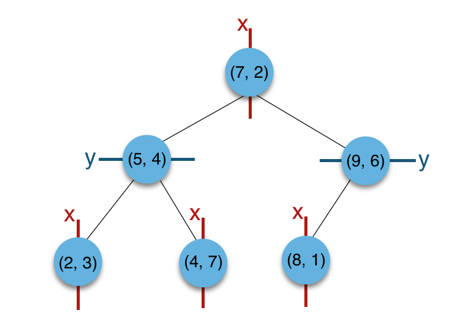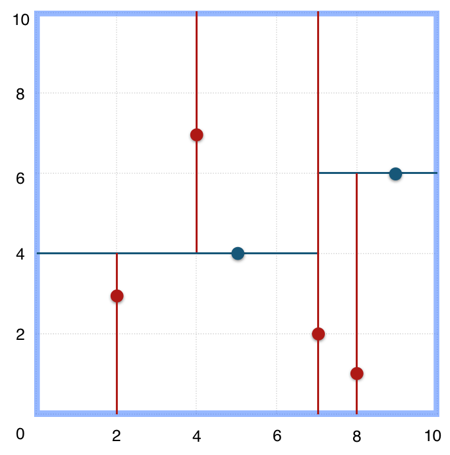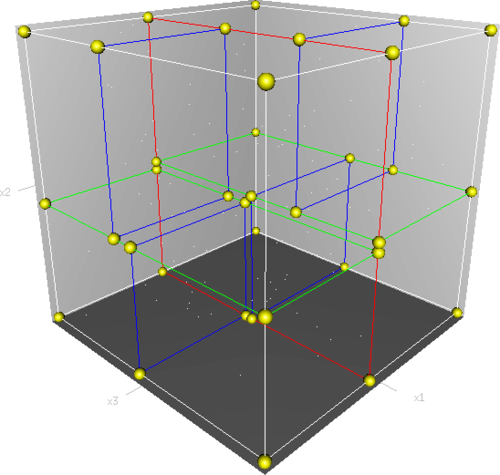def kd_tree(points, depth):
if 0 == len(points):
return None
cutting_dim = depth % len(points)
medium_index = len(points) // 2
points.sort(key=itemgetter(cutting_dim))
node = Node(points[medium_index])
node.left = kd_tree(points[:medium_index], depth + 1)
node.right = kd_tree(points[medium_index + 1:], depth + 1)
return node


1.2）寻找d维最小坐标值点

a）若当前节点的切分维度是d

b）若当前节点的切分维度不是d

def findmin(n, depth, cutting_dim, min):
if min is None:
min = n.location
if n is None:
return min
current_cutting_dim = depth % len(min)
if n.location[cutting_dim] < min[cutting_dim]:
min = n.location
if cutting_dim == current_cutting_dim:
return findmin(n.left, depth + 1, cutting_dim, min)
else:
leftmin = findmin(n.left, depth + 1, cutting_dim, min)
rightmin = findmin(n.right, depth + 1, cutting_dim, min)
if leftmin[cutting_dim] > rightmin[cutting_dim]:
return rightmin
else:
return leftmin


1.3）新增节点

def insert(n, point, depth):
if n is None:
return Node(point)
cutting_dim = depth % len(point)
if point[cutting_dim] < n.location[cutting_dim]:
if n.left is None:
n.left = Node(point)
else:
insert(n.left, point, depth + 1)
else:
if n.right is None:
n.right = Node(point)
else:
insert(n.right, point, depth + 1)


1.4）删除节点

a）无子树

b）有右子树

c）无右子树有左子树

（之所以未采用findmax(T.left, cutting-dim=x)节点来替换被删节点，是由于原被删节点的左子树节点存在x维度最大值相等的情形，这样就破坏了左子树在x分割维度的坐标需小于其根节点的定义）

def delete(n, point, depth):
cutting_dim = depth % len(point)
if n.location == point:
if n.right is not None:
n.location = findmin(n.right, depth + 1, cutting_dim, None)
delete(n.right, n.location, depth + 1)
elif n.left is not None:
n.location = findmin(n.left, depth + 1)
delete(n.left, n.location, depth + 1)
n.right = n.left
n.left = None
else:
n = None
else:
if point[cutting_dim] < n.location[cutting_dim]:
delete(n.left, point, depth + 1)
else:
delete(n.right, point, depth + 1)


2）最近邻搜索

a） 首先从根节点(7,2)出发，将当前最近邻设为(7,2)，对该k-d tree作深度优先遍历。以(3,5)为圆心，其到(7,2)的距离为半径画圆（多维空间为超球面），可以看出(8,1)右侧的区域与该圆不相交，所以(8,1)的右子树全部忽略。

b） 接着走到(7,2)左子树根节点(5,4)，与原最近邻对比距离后，更新当前最近邻为(5,4)。以(3,5)为圆心，其到(5,4)的距离为半径画圆，发现(7,2)右侧的区域与该圆不相交，忽略该侧所有节点，这样(7,2)的整个右子树被标记为已忽略。

c） 遍历完(5,4)的左右叶子节点，发现与当前最优距离相等，不更新最近邻。所以(3,5)的最近邻为(5,4)。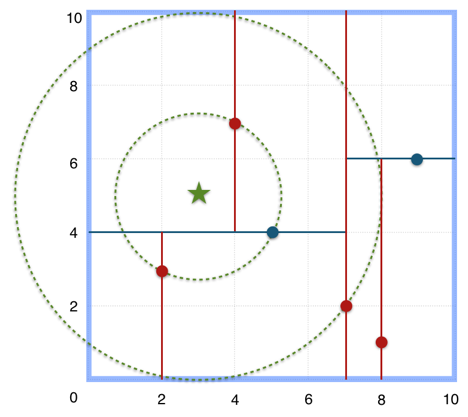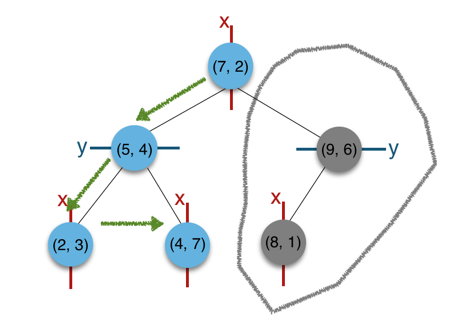3）复杂度分析

操作	平均复杂度	最坏复杂度



4）scikit-learn使用

scikit-learn是一个实用的机器学习类库，其有KDTree的实现。如下例子为直观展示，仅构建了一个二维空间的k-d tree，然后对其作k近邻搜索及指定半径的范围搜索。多维空间的检索，调用方式与此例相差无多。

#!/usr/bin/python
# -*- coding: UTF-8 -*-
import numpy as np
from matplotlib import pyplot as plt
from matplotlib.patches import Circle
from sklearn.neighbors import KDTree

np.random.seed(0)
points = np.random.random((100, 2))
tree = KDTree(points)
point = points

# kNN
dists, indices = tree.query([point], k=3)
print(dists, indices)

print(indices)

fig = plt.figure()